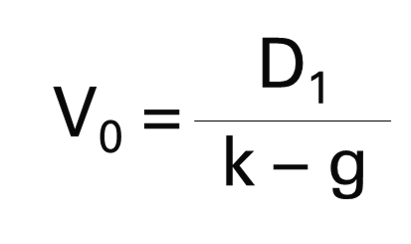top of page# A question of "k": why discount rates are so important to valuation

It can be confusing, what happens on a day like Friday the 5th of July, 2019. The jobs report comes out better than expected and markets go down. To any normal person this seems backwards. To the pundits in financial media it seems normal, but that's probably because they've seen it so often in recent history. They (the pundits) explain how, due to the strong jobs report, the market is now fearful the Federal Reserve will not cut rates. But they do not explain why that occurs.

Here's why, in a nutshell.

## The Cost of Capital - or, "k"

The cost of capital, denoted as k in financial mathematics, is the factor to which asset values are most sensitive. According to the most fundamental valuation equation, Gordon's Growth Model, value today equals the next period's dividend divided by the cost of capital (k) less a growth rate into perpetuity.("r" is sometimes denoted in place of "k" for cost of capital. "Discount rate", "required return", and "cost of capital" are often used interchangeably.)

In reality, valuation is much more nuanced. There is a lot of uncertainty about what earnings will be in the future. While prices are ultimately set by the last trade, valuation methodologies help us approximate a range of what the price should be based on reasonable expectations. The most useful valuation tool, we believe, is the discounted cash flow (DCF) model. Here we assume some level of future cash flows over n years, along with a terminal value in the final year, then sum the present values. Theoretically, if we knew the exact cash flows of each year into the future, we could calculate the precise value today by discounting at the risk-free rate.

Unfortunately we cannot predict the future. Hence, we cannot rely on DCF valuation as the sole, or even primary, tool for choosing stocks. Rather, we use the DCF model to help us understand, all else equal, (1) a reasonable high/low valuation range and (2) how sensitive a firm's valuation is to different discount rates and future growth rates (or levels of free cash flow).

Our aim here is to show:

• (a) the isolated impact to valuation from changes to the discount rate.

• (b) the effect changes in interest rates have on the discount rate.

We use Adobe (ticker: ADBE) as the example - a firm with strong returns on invested capital, low CAPEX needs, and high margins.

We show three scenarios (below), each with a 100bp difference in the discount rate. Within each example we have three valuation estimates [V(e)]: Upper V(e), Median V(e), and Lower V(e). Intuitively, the upper estimate assumes a more aggressive growth rate where the lower estimate assumes a more conservative growth rate. For simplicity, we assume the same cash flows in each example for each of the three valuation estimate sub-scenarios (upper, median, lower).

The discount rate is calculated (for conceptual purposes) as the risk-free rate, typically the 10-year government bond, plus some risk premium.

k = Rf + risk premium(s)

If we assume a 5.0% constant risk premium for ADBE, then we have the following risk-free rates in each scenario:

• Scenario 1: 4.0%

• Scenario 2: 3.0%

• Scenario 3: 2.0%

### Scenario 1

where, k = 9.0% @ t0 and increases linearly to 9.8% terminally

Median V(e) = \$208.12### Scenario 2

where, k = 8.0% @ t0 and increases linearly to 8.7%% terminally

Median V(e) = \$252.75### Scenario 3

where, k = 7.0% @ t0 and increases linearly to 7.7%% terminally

Median V(e) = \$320.81The obvious conclusion is that, all else equal, for every 100 basis points by which the discount rate is reduced, there is a significant jump in fair value - V(e). The disparity between scenarios is striking.

### Median V(e) percent delta from other scenarios

Scenario 1:

• 17.65% lower than Scenario 2

• 35.13% lower than Scenario 3

Scenario 2:

• 21.44% higher than Scenario 1

• 21.22% lower than Scenario 3

Scenario 3:

• 54.15% higher than Scenario 1

• 26.93% higher than Scenario 2

These calculations should be enlightening as to why markets gyrate (and why other asset prices would also gyrate if priced every second). The market is constantly synthesizing an expectation for, among many other things, future interest rates.

One can argue about what the discount rate should be for a given asset and to what extent it should be tied to the risk-free rate. The point of this post is to show the distinct and natural sensitivity. V(e) cannot be separated from k, yet value can never be known with precision because k (or future interest rates) cannot be known with certainty. We can only approximate.

Hence, when the market gets a strong jobs report and counterintuitively goes down, it is because of expected higher future rates. All eyes are locked on central bank policy because of the obvious ramifications to asset prices. The question is, by how much can rates rise without sending asset prices into a tailspin? That's a topic for another day. But my guess is not much. The good news is, for long-term investors with proper risk protections, the market's near-term interest rate obsessions matter less than they might to the average investor. With the right (antifragile) strategy, one is relieved of the stresses of interest rate blunders.

See All
bottom of page